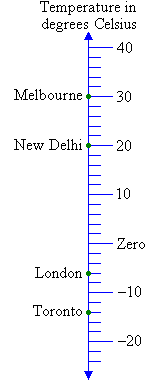Year 8 Interactive Maths - Second Edition

## The Number Line

A number line is a line on which numbers are represented in ascending order.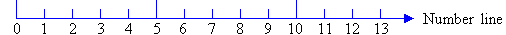This number line can be extended to the left to represent numbers which are smaller than 0.  Such numbers are called negative numbers.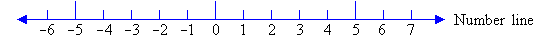For example, –1 (called negative one or minus one) is positioned 1 unit to the left of 0.  So, –1 is less than zero.

Similarly, –2 (called negative two or minus two) is 2 less than zero
and         –3 (called negative three or minus three) is 3 less than zero
and so on.

###### From the preceding discussion we can define the number line as follows:

A line on which the numbers on both sides of zero are represented is said to be a number line (or directed number line).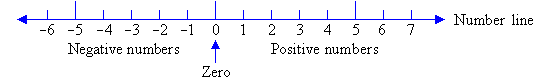Positive numbers are represented on the right of the zero and negative numbers are represented on the left of the zero as shown in the above diagram.

#### Example 1

Write down the elevation information of the following places on a number line.  Use the minus sign (–) to represent elevation below sea level.

a.  Mt. Kosciusko (Australia)     2230 m above sea level
b.  Bangalore (India)     1000 m above sea level
c.  Death Valley (USA)     85 m below sea level
d.  Qattara Depression (Egypt)     392 m below sea level

##### Solution: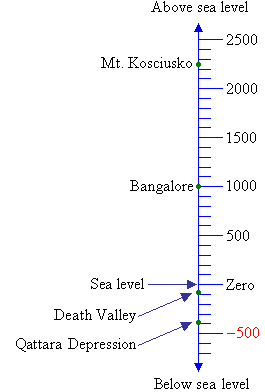#### Example 2

Write down the temperature information for the following places on a number line and use the minus sign (–) to indicate any temperature that is below zero.

b.  Melbourne (Australia)     30ºC
c.  London (UK)     –6ºC
d.  New Delhi (India)     20ºC

##### Solution: